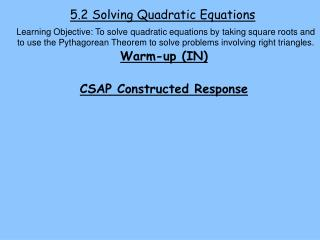DownloadDownload PresentationDownload Presentation- - - - - - - - - - - - - - - - - - - - - - - - - - - E N D - - - - - - - - - - - - - - - - - - - - - - - - - - -
##### Presentation Transcript

1. 5.2 Solving Quadratic Equations Learning Objective: To solve quadratic equations by taking square roots and to use the Pythagorean Theorem to solve problems involving right triangles. Warm-up (IN) CSAP Constructed Response

2. Learning Objective: To solve quadratic equations by taking square roots and to use the Pythagorean Theorem to solve problems involving right triangles. Notes Properties of Square roots ** Product property - ** Quotient property -

3. Learning Objective: To solve quadratic equations by taking square roots and to use the Pythagorean Theorem to solve problems involving right triangles. EX 1 – Solve SADMEP! Try t his p. 282 (middle and bottom)

4. Learning Objective: To solve quadratic equations by taking square roots and to use the Pythagorean Theorem to solve problems involving right triangles. EX 2 – A helicopter 85 ft above a designated area drops a boxes of food to a disaster area. The height of the box above the ground can be modeled by , where t is the time in seconds after it has been released. After how many seconds will it hit the ground?

5. Learning Objective: To solve quadratic equations by taking square roots and to use the Pythagorean Theorem to solve problems involving right triangles. c a b 8.4 B A a. 5.3 b C Pythagorean Theorem! In a right , the sum of the squares of the lengths of the legs is equal to the square of the hypotenuse. EX 3 – Find the unknown length

6. Learning Objective: To solve quadratic equations by taking square roots and to use the Pythagorean Theorem to solve problems involving right triangles. 1.9 J b. K k 2.8 L

7. Learning Objective: To solve quadratic equations by taking square roots and to use the Pythagorean Theorem to solve problems involving right triangles. S 106 ft 75 ft P Q R EX 4 – What must be the length of QS so that it contacts the ground halfway between the base of the tower and the point where PS contacts the ground?

8. Out – Describe the procedure you would use to solve Summary – So, I was thinking… POW!! HW – p. 287 #21-43 odd, 51-54